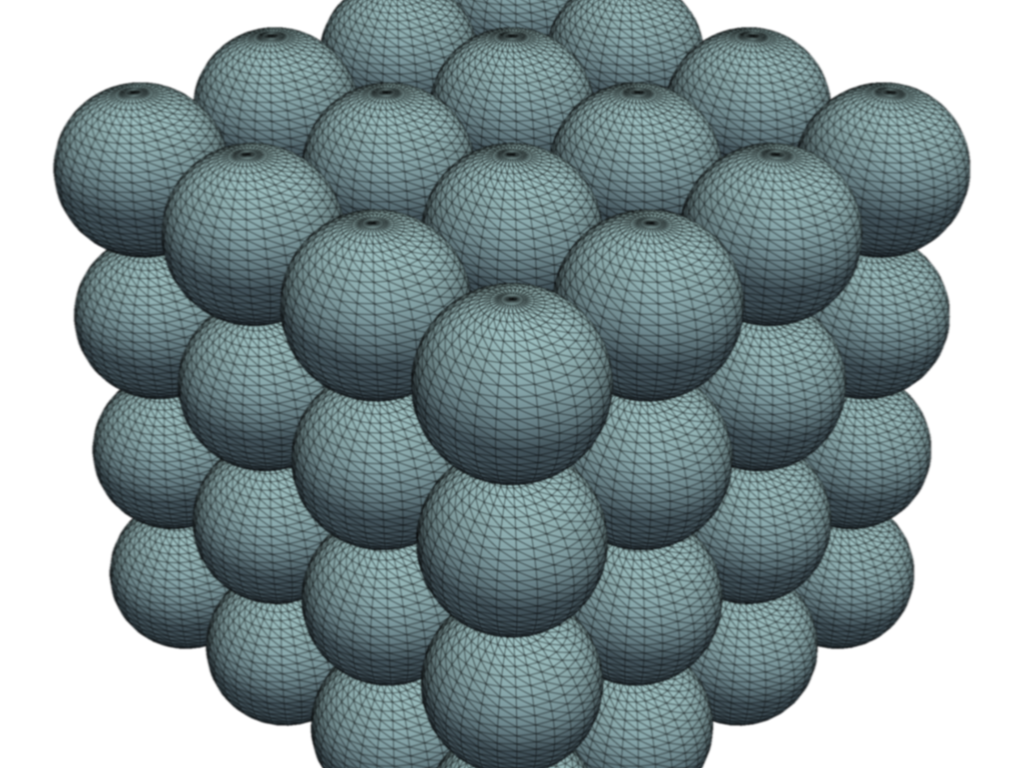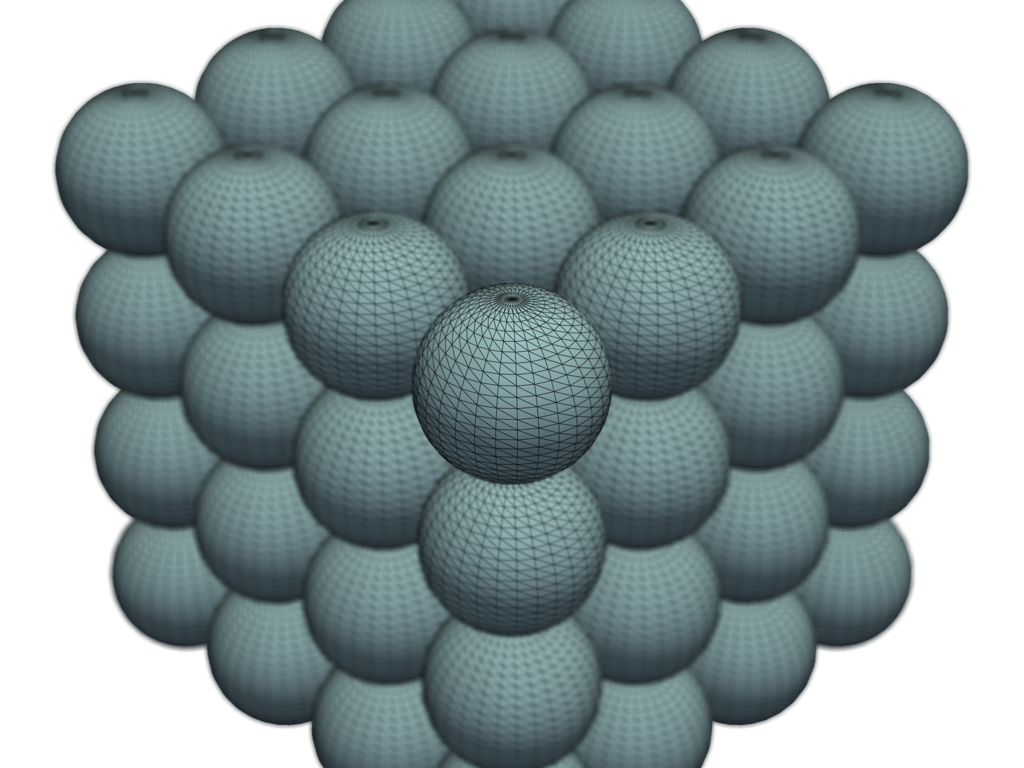# Blurring#

This example shows how you can use `add_blurring` to blur a plot, or use `enable_depth_of_field` to highlight part of your plot.

```import pyvista as pv
```

# Create several spheres#

```# We use a uniform grid here simply to create equidistantly spaced points for
# our glyph filter
grid = pv.UniformGrid(dimensions=(4, 4, 4), spacing=(1, 1, 1))

spheres = grid.glyph(geom=pv.Sphere(), scale=False, orient=False)
```

# Blur the plot#

Add a few blur passes to blur the plot

```pl = pv.Plotter()
pl.camera.zoom(1.5)
pl.enable_anti_aliasing('ssaa')
pl.show()
```Note how this is different than selectively blurring part of the mesh behind the focal plane

```pl = pv.Plotter()